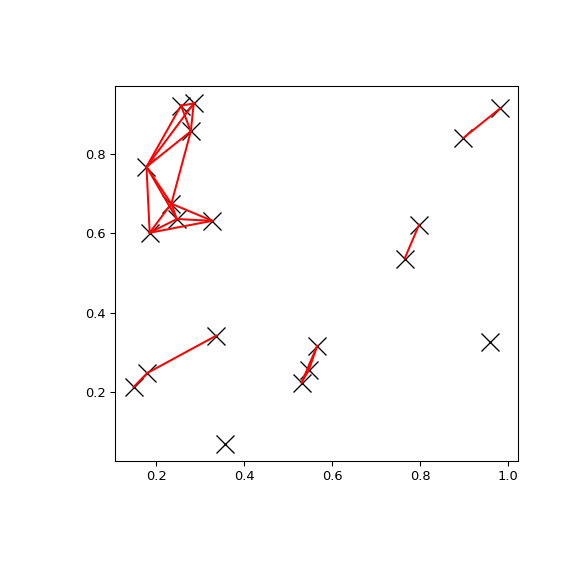# scipy.spatial.cKDTree.query_pairs¶

cKDTree.query_pairs(self, r, p=2.0, eps=0)

Find all pairs of points in self whose distance is at most r.

Parameters
rpositive float

The maximum distance.

pfloat, optional

Which Minkowski norm to use. p has to meet the condition 1 <= p <= infinity. A finite large p may cause a ValueError if overflow can occur.

epsfloat, optional

Approximate search. Branches of the tree are not explored if their nearest points are further than r/(1+eps), and branches are added in bulk if their furthest points are nearer than r * (1+eps). eps has to be non-negative.

output_typestring, optional

Choose the output container, ‘set’ or ‘ndarray’. Default: ‘set’

Returns
resultsset or ndarray

Set of pairs (i,j), with i < j, for which the corresponding positions are close. If output_type is ‘ndarray’, an ndarry is returned instead of a set.

Examples

You can search all pairs of points in a kd-tree within a distance:

>>> import matplotlib.pyplot as plt
>>> import numpy as np
>>> from scipy.spatial import cKDTree
>>> np.random.seed(21701)
>>> points = np.random.random((20, 2))
>>> plt.figure(figsize=(6, 6))
>>> plt.plot(points[:, 0], points[:, 1], "xk", markersize=14)
>>> kd_tree = cKDTree(points)
>>> pairs = kd_tree.query_pairs(r=0.2)
>>> for (i, j) in pairs:
...     plt.plot([points[i, 0], points[j, 0]],
...             [points[i, 1], points[j, 1]], "-r")
>>> plt.show()#### Previous topic

scipy.spatial.cKDTree.query_ball_tree

#### Next topic

scipy.spatial.cKDTree.sparse_distance_matrix